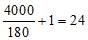Bar Bending Schedle

# Bar Bending Schedule for Reinforcement Beam

## Bar Bending Schedule for Reinforced Concrete Beam

Bar bending schedule provides the RCC Calculation for reinforced concrete beam. It provides details of the reinforcement cutting length, type of bends & bend length. We will take 1 example for reinforcement QTY calculations for a Concrete Beam.### Example of the Beam Reinforcement Calculation.

Consider a beam of clear length of 4-m, 300-mm wide by 450-mm depth. It consists of 2-12 diameter bars at top, & 2-16 diameter & 1 – 12 diameter bars at the bottom. Diameter of stirrup is 8-mm spaced at 180-mm center to center. Clear cover to reinforcement provided is 40-mm.Now we will calculate the length of the reinforcement based on shapes of the reinforcement required for reinforced concrete beam in above example. We will start with bottom reinforcement, B-1. Bar shape of B-1 is as shown below.### Bar Bending Schedule for RCC Slab With Complete Details

Length of B-1 = Clear distance between walls + two x width of walls – two x bar cover + two x bend length Bend length = Six x 16 = 96 consider as 100-mm Bend length is calculated as 6 x diameter of the bar for reinforcement conforming to IS: 1786 -1961 Length of B-1 = 4000 + two x 230 – two x 40 + 2 x100 = 4580-mm Length of bar B-2 is calculated based on the shape of this bar. This bar bends up near the support as shown below.Length of bar B-2: A + B + C = 4000 + two x 230 – two x 40 + (1.414xH – H) H = 450 – two x 40 – two x 12 – two x 12/2 = 334-mm B-2 = 4000 + 2 x 230 – two x 40 + (1.414 x 334 – 334) = 4518.3 = 4520-mm Length of the Bar T-1 = 4000 + two x 230 – two x 40 = 4380-mm Length of Stirrups S-1:Stirrups are spaced at 180-mm C/C . Stirrups are provided between walls or support for the beam. No. of stirrups required for given beam =Length a = 450 – two  x 40 – 8 = 362-mm Length b = 300 – two x 40 – 8 = 212-mm Therefore, length of one stirrup S1 = two x ( 212 + 362 + 90 ) = 1328-mm Where 90-mm is the minimum hook length as per IS 2502 – Table – II.

Thank You.